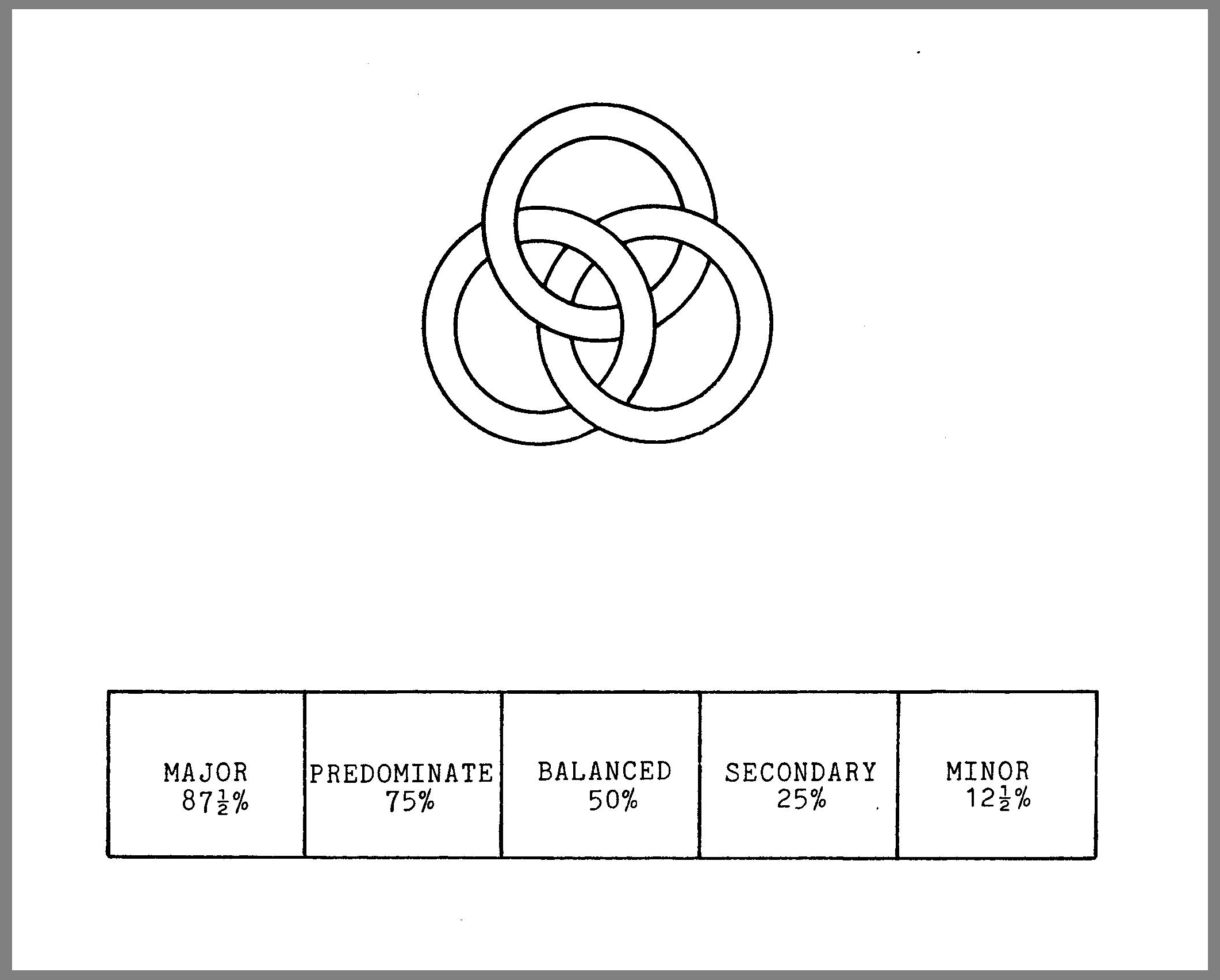# Diagram 009

### Re talks:  84Diagram # 009 illustration

I have used certain terminology, referring to the First, Second and Third forces.  But if you have continued to think of them that way, you must stop it.  There is no such thing as First, Second or Third force floating around.  One reason I am changing the terminology is that the numerical value I originally assigned did not have anything intrinsic in its order.  First force is no better than Third force; Third force is not worth more numerically than First or Second force.

Here is new way to think about the interaction between the forces:  What it amounts to — as pictured in this drawing — is a series of vivus seams — living juncture points where the forces connect.  If you think of each ring as representing one of the forces, each is interconnected with the other two and these seams are alive at the points where the forces are joined.

The forces’ interaction is a matter of degree.  If we were describing two of the Three Forces, there would be one place where their interaction would be symmetrical; that is, each force would be contributing one-half.  (The total amount of energy which could turn into form would be 100%)  That is the middle stage which I have marked “50% — 50%”.  Consider — simply for the sake of this map — that one side is positive and the other negative.  (Of course, when the two forces are actually joined, it is irrelevant to say that one is positive and one is negative.)

On this map the extreme ends represent interactions wherein things are turned in such a way that one of the forces is the major force.  These are marked “87 1/2% — 12 1/2%”.

This map has the potential to help you begin to See what makes things operate.  It is never simply a head to head confrontation or simple dichotomy between two forces.  The action of one force could range from 99% to 1%. Do you See that at the extreme — if First force were contributing 99% and Second force 1% — there would be a momentum to one side and things would move in a particular direction?  Of course, for the sake of description we are ignoring Third force, which makes this alive.  There is no such thing as 100%; that is, the forces do not exist independently.

Continuing the last example, suppose that things shifted so that First force was contributing 75% of the total transfer of energy, rather than 99%.  Things would not be moving at quite the same momentum because the force producing the primary momentum would not be as strong.  Then if the percent shifts again so that the two forces are in a symmetrical relationship, the situation is entirely different.

Remember that I do not invent maps for the sake of inventing maps.  This one is as disposable as any other.  Still, what I am describing to you is astounding.  The continual shifting of forces is what causes things to operate as they do.  It is a relative matter of these three rings — using this map for the time being — moving nonstop.